Bitcoin Energy-Value Equivalence

 Crypto Words has moved! The project has migrated to a new domain. All future development will be at WORDS.

Bitcoin Energy-Value Equivalence

Take-aways

• Energy, raw Joules alone, can be used to estimate Bitcoin’s fair value
• Increased energy input increases the fair value of a Bitcoin (and vice versa for decreases)
• Bitcoin’s price is mean reverting to its Energy Value
• The Energy Value model states that if all miners were to stop mining Bitcoin tomorrow, the power input would be zero and Bitcoin would be worthless
• The Energy Value formula says that Bitcoin has a fair value of approximately \$11,500 today (12 December 2019), 50% higher than the current trading price.

Bitcoin Energy-Value Equivalence

In Bitcoin’s Production Cost we observed the relationship between Bitcoin’s Price and Bitcoin Mining expenditure. Variations in Bitcoin’s Production Cost were found to be primarily driven by the level of electrical energy input and energy efficiency of mining hardware, with many other factors assumed constant.

This begs the question:

Can the fundamental value of Bitcoin be accounted for by raw energy alone?

A Fair Value for Bitcoin

The hypothesis:

Bitcoin’s fair value is a function of energy input, supply growth rate and a constant representing the fiat dollar value of energy.

These variables can be combined into the following equation, termed Bitcoin’s Energy Value (V):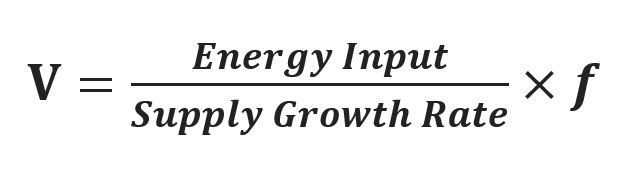The Energy Value Formula

Where:

• Energy Input (unit: Watts) = Hash Rate (GH/s) * Mining Energy Efficiency (J/GH)
• Supply Growth Rate (unit: s-1) = Annual increase in circulating Bitcoins, equivalent to the inverse of Stock-to-Flow. Calculated as the annual rate (unit: year-1) of change in circulating Bitcoins and then converted to seconds
• Fiat Factor (\$USD/Joule) = A constant conversion factor to allow for the fiat USD value of energy

As all units of hash rate and supply rate cancel out, this equation suggests that the fair value of Bitcoin (V) can be represented as a function of the Joules of energy spent to produce it: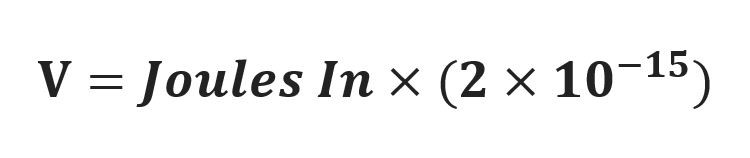The Energy Value Formula: Bitcoin’s fair value is a function of Joules

Building Bitcoin’s Energy Value

To test the theory all input data, except for mining Energy Efficiency, was sourced from Blockchain.info.

The challenging piece of the puzzle is obtaining a good estimate for Bitcoin’s Energy Efficiency through time.

Estimating Bitcoin Mining Energy Efficiency (J/GH)

The power required to fuel Bitcoin mining is driven by two parts, the hash rate to solve the SHA-256 algorithm and the energy efficiency of the mining hardware itself. In its early years, Bitcoin was mined on very electrically inefficient CPUs and GPUs.

The current era ASICs have energy efficiencies over 100,000 times greater than the average Bitcoin mining hardware of 2009. This means that a higher relative portion of the average miner’s electrical bill today is efficiently converted into hashing power.

To estimate a historic profile of Bitcoin mining hardware Energy Efficiency, the efficiency rates for 150 Bitcoin hardware models from Cambridge (ASICs only), BitcoinWiki (FPGAs) and Bitcoin.it (ASICs, CPUs and GPUs) were collated. All ASICs, FPGAs and Intel, AMD and Nvidia hardware were considered where Energy Efficiency (J/GH) was provided and where an estimated hardware release date was found. Common models were grouped and the average energy efficiency for that model calculated on an equal weight basis.

The daily energy efficiency of the Bitcoin network was then calculated as the equally weighted average of all hardware which was within 2 years of its release for CPUs/GPUs/FPGAs and within 1.5 years of its release for ASICs. This difference in depreciable lifespan was chosen because:

• The hardware within model groups for CPUs and GPUs generally span several years
• Bitcoin mining was generally less competitive in its early years
• Other research also suggests a 1.5 year depreciation lifespan is typical for ASICs in more recent years

Finally, a 1 month moving average of Energy Efficiency was calculated to allow for the phase-in and phase-out of model types.

In reality, some hardware models had wider usage and some longer lifespans. However, at risk of increasing the historical error in the Energy Value model and in attempt to produce an unbiased outcome, no other hardware exclusion logic, data cleansing or data manipulation was conducted.

The above process yields the following Bitcoin energy efficiency profile (Joules / Giga Hash) over time.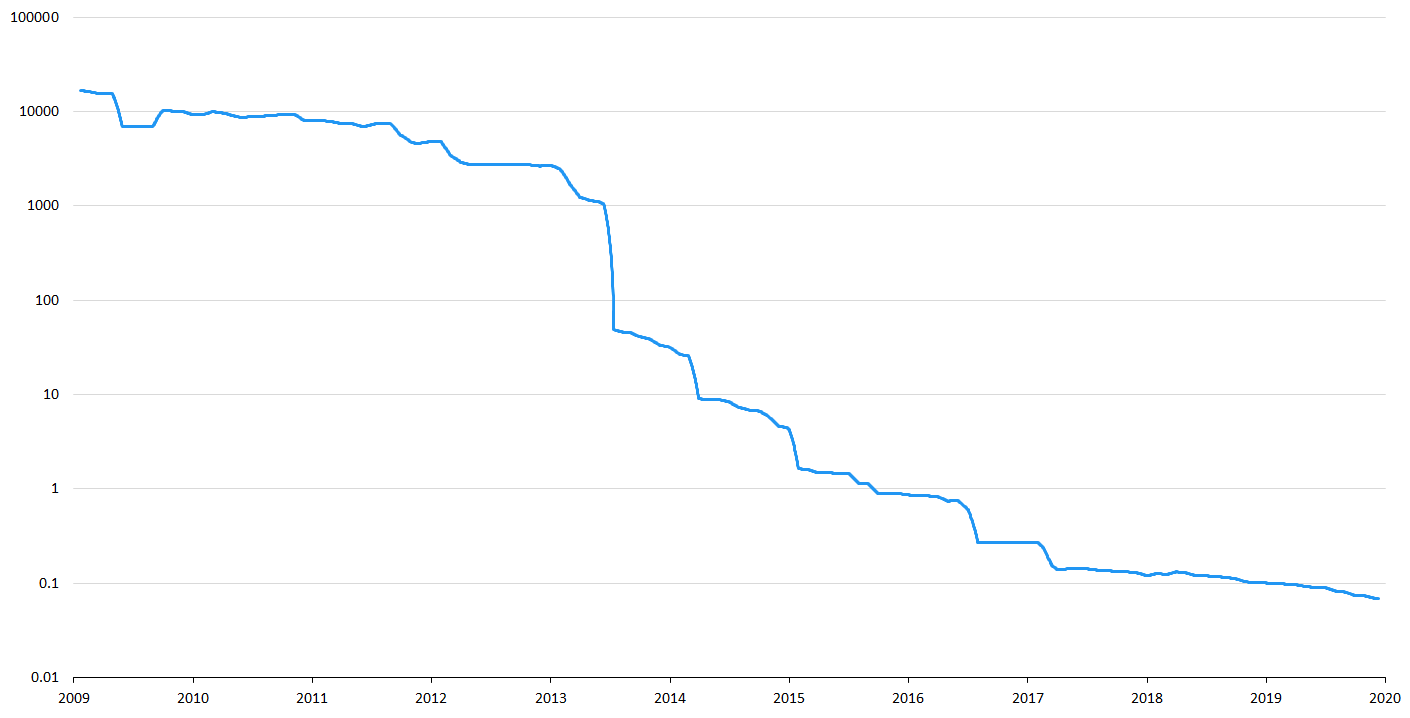The S-curve of increasing Bitcoin Energy Efficiency (represented by a falling J/GH over time). Note the sharp increase in efficiency from the introduction of ASICs through 2013 and 2014.

Fiat Factor Constant (\$/J)

The Fiat Factor is a necessary constant to convert the units of energy input (Joules) to fiat currency US Dollars. It is simply a representation of how much “we” value energy.

Based on the Energy Efficiency profile above, the resulting Fiat Factor is:

2.0E-15

The Fiat Factor value is dependent on the accuracy of the Energy Efficiency profile and therefore the value provided here should be considered a close estimate. In the long term, a declining US Dollar, hyperinflation or fiat currency collapse would result in the Fiat Factor increasing in a 1-for-1 relative manner.

The Result

Plotting the result shows a visually strong fit for the Bitcoin Energy Value to historic price.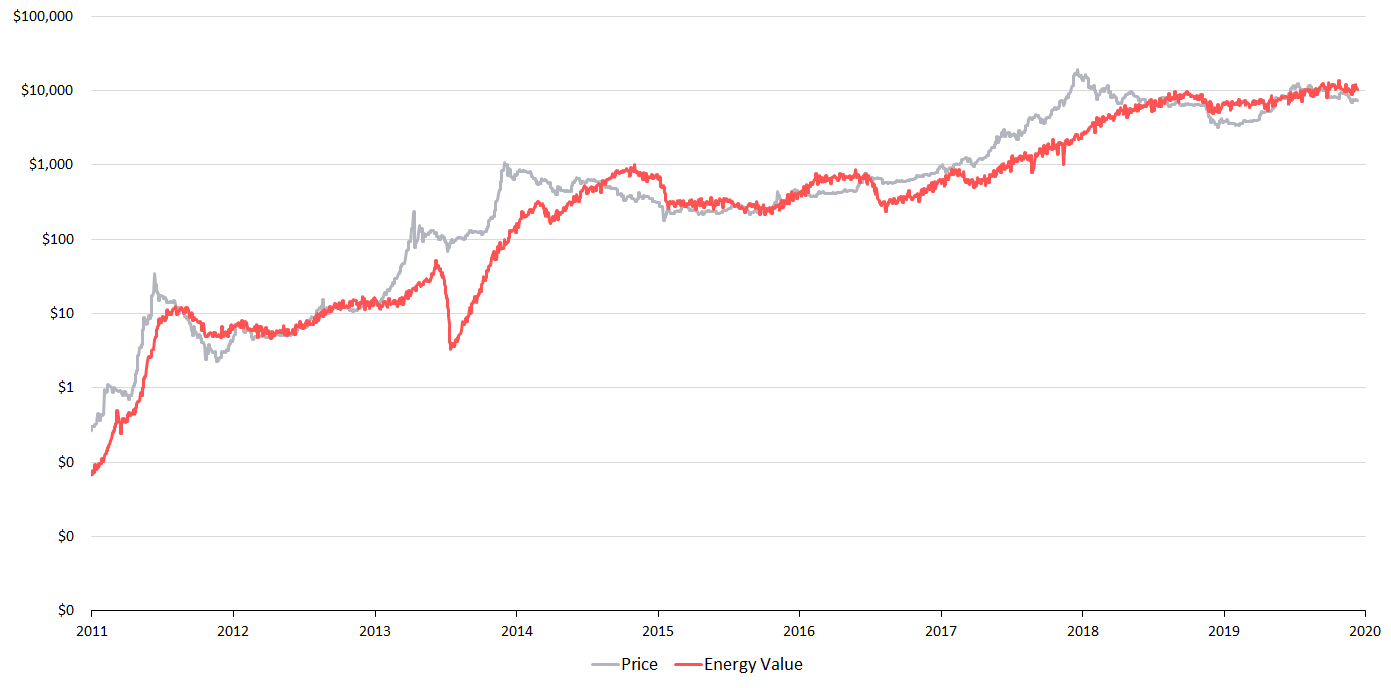10 Years of Bitcoin’s Energy Value

The plunge in Bitcoin’s energy value in 2013/14 is largely driven by the transition from GPU/FPGA to ASIC hardware. While it is likely there was a substantial drop during this period, it may be somewhat exaggerated here due to the hardware usage and depreciation model assumptions outlined above.

Market Forces — Bridging Supply and Demand

The first question is, does a Bitcoin Energy Value make logical sense?

In “Modeling Bitcoin’s Value with Scarcity”, Plan B found a strong relationship between market price and the scarcity of Bitcoin and other hard assets. We can posit that this represents the fundamental relationship of human “demand” for hard, value preserving assets over time.

But there’s a catch.

Not all scarce assets have nor preserve wide-spread market value. For example, there are approximately 3,000 cryptocurrencies with market caps under \$500. Many of these coins have “constrained” supply models, but they have been assessed by the market as having no fundamental value. Scarce, but not valuable. The same can be said for bad art, bananas stuck to walls and many other rare and unique throw-away assets. This makes sense, as scarce assets which are easy to acquire or replicate typically have low market value.

Consistent, high levels of human effort are generally linked with demand.

When Energy is dedicated to a task, the supplier of energy (the worker) expects there to be a demand for their effort. When a supplier sees growing demand for the fruits of their labour, they will work harder in attempt to reap greater benefits. Others will likely also contribute to capture some reward. However, should demand for a supplier’s labour fall, or should the opportunity decline to a point at which they can achieve a better return elsewhere, the supplier will likely cease committing energy to that task.

This is exactly how the war for Bitcoin’s hash rate has been fought, and this is the argument for Bitcoin’s Energy Value.

Consistent energy input represents a balance between supply and demand. Rising market prices incentivize increased energy input via hash power growth and technology improvements which result in greater energy efficiencies. For this reason, great increases in market price typically result in long-term increases in committed energy and therefore increases in Bitcoin’s Energy Value. However, when speculation causes skyrocketing prices, without a corresponding increase in energy input, price has historically collapsed back to the Energy Value.

It is mean reverting phenomenon. As would be expected with any intrinsic value estimate driven from fundamentals.

Bitcoin’s price and Energy Value tend towards each other, they are like magnets. While deviations between the two can and do exist, they have always closed. Despite being mathematically independent to Bitcoin’s trading price and volume, Energy Value is connected by the invisible hand of the market.

By capturing long-term demand for scarce assets based on supply growth rate (stock-to-flow) and energy input, Energy Value represents the symbiotic relationship between Bitcoin supply and demand.

Performance

On daily data from January 2010, the Energy Value formula has a R2 to the actual Bitcoin price of 80% (the higher the R2, the better the model fits reality). By comparison, the stock-to-flow model has a R2 of 88% on the same data. While 8% less than the stock-to-flow model, there are a few things to consider:

• Bitcoin’s Energy Value is highly dependent on the estimated Energy Efficiency. For this analysis, 150 Bitcoin hardware details were manually collated, there is possibility of data or omission error. The depreciation periods are approximations of reality. Efficiency can also vary depending on operating conditions and overclocking. It is not possible to get a perfect representation of what hardware every Bitcoin miner is using at every point in time through history. Hence, some error here is expected.
• If all Bitcoin miners were to suddenly cease mining Bitcoin, stock-to-flow would predict a Bitcoin price of infinity. The Energy Value predicts zero. Should all miners abandon Bitcoin — which could occur via a catastrophic event (such as the breaking of the SHA-256 algorithm), through the creation of a “better” money / store of wealth — no new blocks would be created, no transactions would be sent and the network would be defunct. Under such a circumstance, the stock-to-flow model alone (0.4*SF³) would assess Bitcoin as having infinite value. The Energy Value model states that if all miners were to stop mining Bitcoin tomorrow, the power input would be zero and Bitcoin would be worthless.
• The stock-to-flow model is a fitted power law. Bitcoin’s price has had exponential performance and the stock-to-flow model’s power law was chosen specifically to match this. By optimising the parameters, a good accuracy is achieved to fit Bitcoins price. The Energy Value has no curve fitting parameters, just one fixed constant to allow for the conversion of pure energy to dollars. In fact, it is likely that the exponential increase in mining hardware power efficiency coupled with the growth in hash rates explains the stock-to-flow’s exponential relationship.

With consideration of the above, an 80% R2 is considered a strong result for the Energy Value.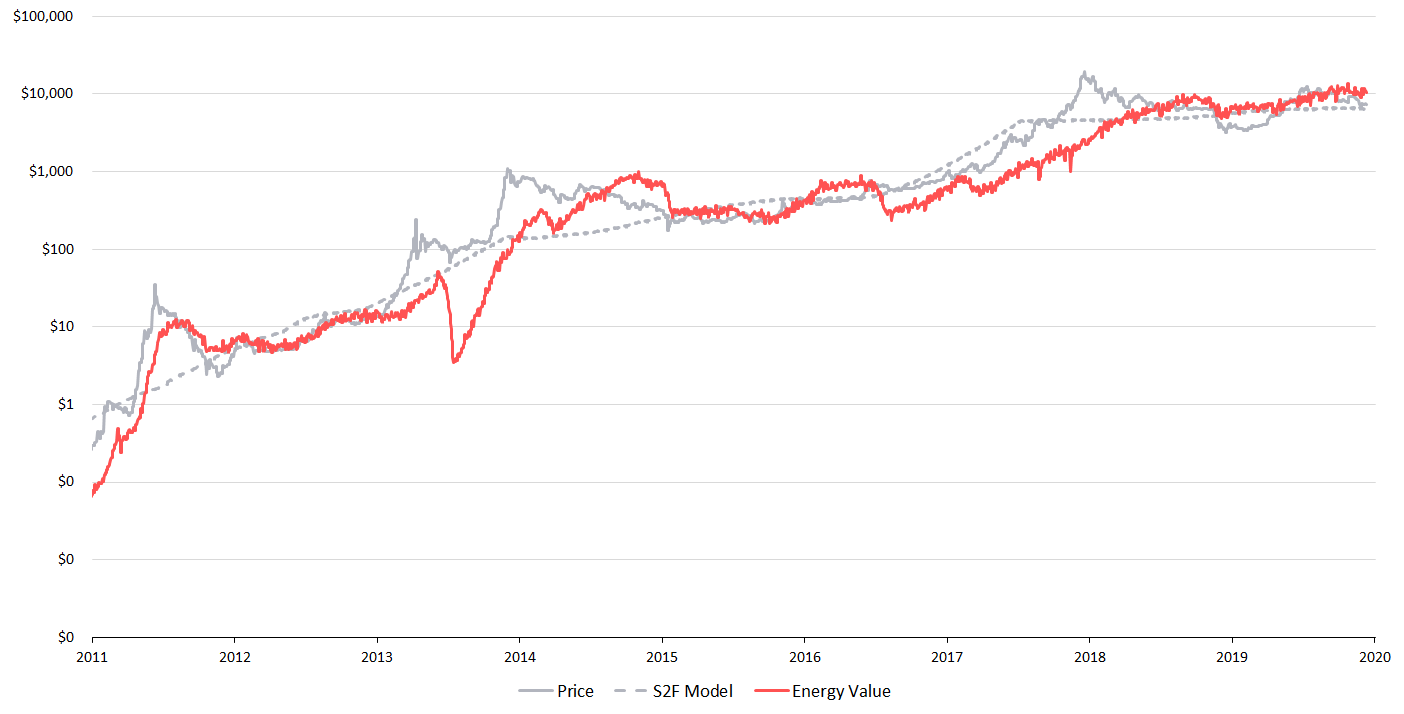Bitcoin’s Energy Value and Stock-to-Flow

Speculation

From the above figure we can see that turning points and wide gaps between Bitcoin’s price and the fair value can signify great times to buy and sell Bitcoin.

Sharp declines in energy input often signify good times to exit the market and strong energy input growth has represented great times to buy

The Energy Value formula says that Bitcoin has a fair value of approximately \$11,500 today (12 December 2019), 50% higher than the current trading price.

This suggests Bitcoin has a great risk-reward in early December 2019. A positive picture is also presented when looking at the below Energy Value Oscillator.

However, energy input can fall at any time.

Historically buying into falling hash rates has been inadvisable, far better risk-reward outcomes are achieved on hash rate recovery.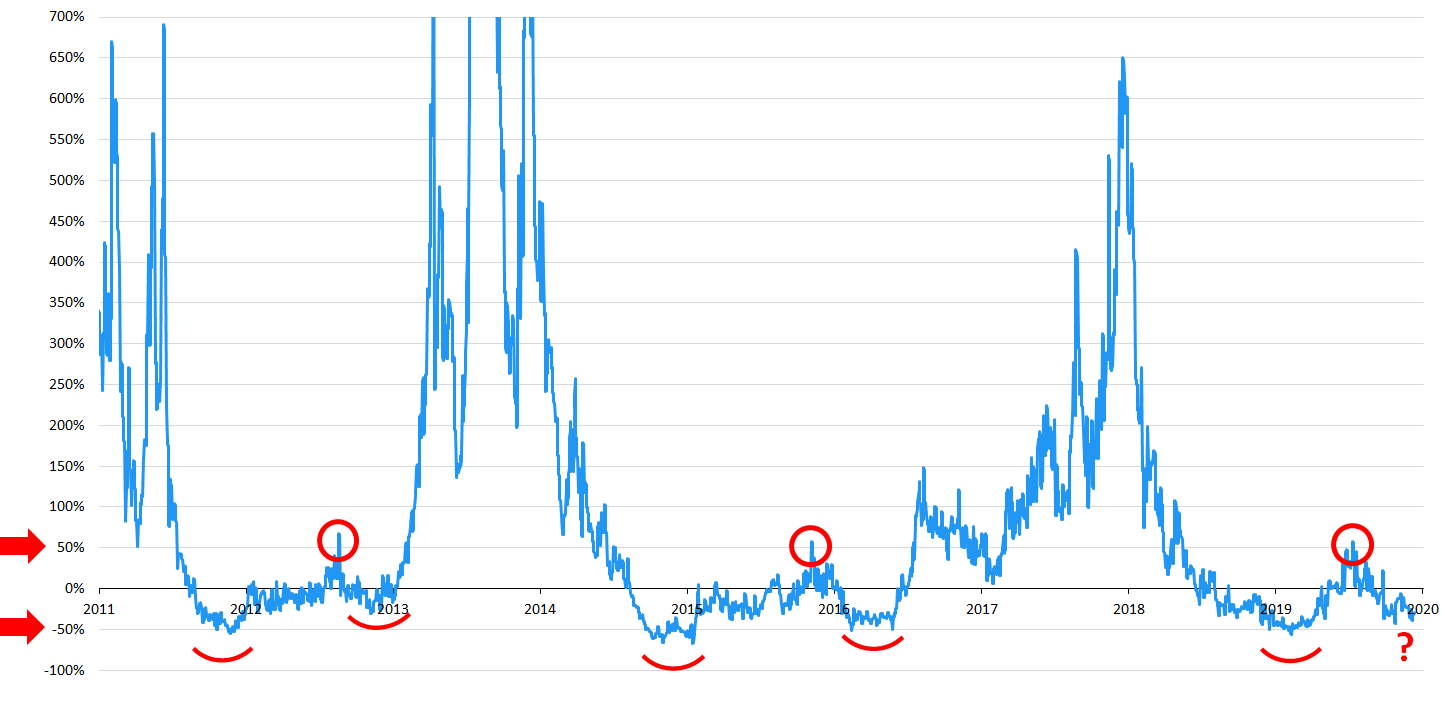Energy Value Oscillator Price as a Percentage of Energy Value. 2019 looks very similar to prior bull run starting characteristics.

Implications

By considering energy and supply growth, we have found an intrinsic link between Bitcoin’s price and its value.

The value of Bitcoin is a function of its energy input in Joules.

Following are some of the implications of the Energy Value formula:

• The health of the mining network is intrinsically linked to Bitcoin’s value
• Increases in electrical energy input will increase the fundamental value of Bitcoin (and vice versa for decreases)
• Higher hash rates (with unchanged energy efficiency) mean each Bitcoin is worth more
• Major improvements in hashing technology (such as the introduction in ASICs) cause considerable volatility in the short-term intrinsic value of Bitcoin due to significant increases in energy efficiency which are not compensated for by equal increases in hash rate growth
• Should quantum computing (or other major technological advancement) require less total network energy to solve the SHA-256 algorithm, the Energy Value formula says Bitcoin’s intrinsic value will fall
• A change to the Bitcoin code which increases Bitcoin’s supply growth rate would decrease the fundamental value of each circulating coin
• In 2140, if Bitcoin is still being hashed, its supply growth rate will be zero. The Energy Value formula, like stock-to-flow, predicts an infinite value for Bitcoin (in USD terms) at that point. However, unlike stock-to-flow, this hinges on the criteria that mining activity is ongoing

If Bitcoin is successfully mass adopted as a store of wealth and/or global currency we may have financial market evidence that value is intrinsically linked to effort, the Joules of energy spent in work.

As humans, our time is limited — it’s our most valuable resource. What we choose to put our energy into, and therefore our time into, is our most valuable choice.

Bitcoin values energy.

Just as mass can be represented by energy, so can Bitcoin’s Price.